## A red candle is 8 inches tall and burns at a rate of 7/10 inch per hour.A blue candle is 6 inches tall and burns at a rate of 1/5 inch per h

Question

A red candle is 8 inches tall and burns at a rate of 7/10 inch per hour.A blue candle is 6 inches tall and burns at a rate of 1/5 inch per hour. After how many hours will both candles be the same hight?

in progress 0
5 months 2021-08-14T01:35:18+00:00 1 Answers 454 views 0

4 hours

Step-by-step explanation:

Given that:

Initial height of red candle = 8 inches

Rate of burning of red candle =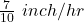Initial height of blue candle = 6 inches

Rate of burning of blue candle =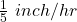To find:

Time taken in hours such that both the candles have the same height.

Solution:

Let the time taken such that both the candles have the same height =hours

Height of red candle afterhours =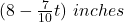Height of blue candle afterhours =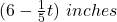Writing both the expressions as equal: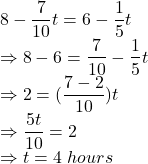After 4 hours, height of both the candles will be same.